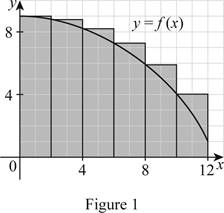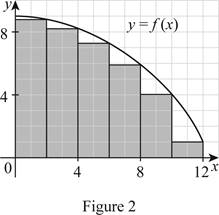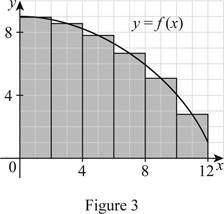# The upper estimate of the area under the graph using six rectangles.### Single Variable Calculus: Concepts...

4th Edition
James Stewart
Publisher: Cengage Learning
ISBN: 9781337687805### Single Variable Calculus: Concepts...

4th Edition
James Stewart
Publisher: Cengage Learning
ISBN: 9781337687805

#### Solutions

Chapter 5.1, Problem 2E

(a)

(i)

To determine

## The upper estimate of the area under the graph using six rectangles.

Expert Solution

The upper estimate of the area under the graph using six rectangles is 86.6.

### Explanation of Solution

Given information:

The curve as y=f(x).

The region lies between x=0 and x=12. So the limits are a=0 and b=12.

Number of rectangles n=6.

The sample points are the right endpoints for the lower estimate, the left end points for the upper estimate, and the mid end points for the mid estimate.

Calculation:

The expression to find upper estimate of areas of n rectangles (Ln) is shown below:

Ln=f(x0)Δx+f(x1)Δx+...+f(xn1)Δx (1)

Here, the left endpoint height of the first rectangle is f(x0), the width is Δx, the height of the left endpoint of the second rectangle is f(x1), and the left endpoint height of the nth rectangle is f(xn1).

Find the width (Δx) using the relation:

Δx=ban (2)

Here, the upper limit is b, the lower limit is a, and the number of rectangles is n.

Substitute 12 for b, 0 for a and 6 for n in Equation (2).

Δx=1206=2

Draw six rectangles using left endpoints as shown in Figure (1).Refer to Figure (1),

Take the left endpoint height of the first rectangle f(x0) value as 9, the left endpoint height of the second rectangle f(x1) value as 8.8, the left endpoint height of the third rectangle f(x2) value as 8.2, the left endpoint height of the fourth rectangle f(x3) value as 7.3, the left endpoint height of the fifth rectangle f(x4) value as 5.9, and the left endpoint f(x5) value as 4.1.

Substitute 6 for n, 9 for f(x0), 2 for Δx, 8.8 for f(x1), 8.2 for f(x2), 7.3 for f(x3), 5.9 for f(x4), and 4.1 for f(x5) in Equation (1).

L6=(9×2)+(8.8×2)+(8.2×2)+(7.3×2)+(5.9×2)+(4.1×2)=18+17.6+16.4+14.6+11.8+8.2=86.6

Therefore, the upper estimate using the left endpoints for n=6 is 86.6.

(ii)

To determine

### The lower estimate of the area under the graph using six rectangles.

Expert Solution

The lower estimate of the area under the graph using six rectangles is 71.

### Explanation of Solution

Draw six rectangles using the right endpoints as shown in Figure (2).The expression to find the lower estimate of the areas of 6 rectangles (R6) is shown below:

R6=L6+f(x6)Δxf(x1)Δx (3)

Here, the upper estimate using the left endpoints for n=6 is L6, the right endpoint height of the right lowermost rectangle is f(x6), and the right endpoint height of the left uppermost rectangle is f(x1).

Refer to Figure (2).

Take the right endpoint height of the left uppermost rectangle f(x1) value as 8.8 and the right endpoint height of the left uppermost rectangle f(x6) value as 1.

Substitute 86.6 for L6, 1 for f(x6), 2 for Δx, and 8.8 for f(x1) in Equation (3).

R6=L6+f(x6)Δxf(x1)Δx=86.6+(1×2)(8.8×2)=86.6+217.6=71

Therefore, the lower estimate using the left endpoints for n=6 is 71.

(iii)

To determine

### The mid estimate of the area under the graph using six rectangles.

Expert Solution

The mid estimate of the area under the graph using six rectangles is 79.6.

### Explanation of Solution

The expression to find mid estimate of the areas of n rectangles (Mn) is shown below:

Mn=f(x1)Δx+f(x2)Δx+...+f(xn)Δx (4)

Here, the mid height of the first rectangle is f(x1), the mid height of the second rectangle is f(x2), and the mid height of the nth rectangle is f(xn).

Draw six rectangles using mid endpoints as shown in Figure (3).Refer to Figure (3).

Take the mid height of the first rectangle f(x1) value as 9, the mid height of the second rectangle f(x2) value as 8.5, the mid height of the third rectangle f(x3) value as 7.8, the mid height of the fourth rectangle f(x4) value as 6.6, the mid height of the fifth rectangle f(x5) value as 5.1, and the mid height of the sixth rectangle f(x6) value as 2.8.

Substitute 6 for n, 9 for f(x1), 2 for Δx, 8.5 for f(x2), 7.8 for f(x3), 6.6 for f(x4), 5.1 for f(x5) and 2.8 for f(x6) in Equation (4).

R6=(9×2)+(8.5×2)+(7.8×2)+(6.6×2)+(5.1×2)+(2.8×2)=18+17+15.6+13.2+10.2+5.6=79.6

Therefore, the mid estimate using mid endpoints for n=6 is 79.6.

(b)

To determine

### Whether L6 is an underestimate or an overestimate.

Expert Solution

L6 is an overestimate of the true area.

### Explanation of Solution

Refer to part (i).

The function y=f(x) shows a decreasing curve.

The upper estimate L6 obtained using the left end points is an overestimate of the true area.

Hence, the upper estimate L6 is an overestimate of the true area.

(c)

To determine

### Whether R6 is an underestimate or an overestimate.

Expert Solution

R6 is an underestimate of the true area.

### Explanation of Solution

Refer to part (ii),

The curve is a decreasing curve.

The lower estimate R6 is obtained using right end points and is an underestimate of the true area.

Hence, the lower estimate R6 is an underestimate of the true area.

(d)

To determine

### The best estimate.

Expert Solution

M6 is the best estimate.

### Explanation of Solution

Refer to part (b) and part (c).

The upper estimate is an overestimate of the true area and the lower estimate is an underestimating of the true area.

Refer to Figure (3).

The mid estimate of the area using mid end points shows the area of each rectangle which appears closer to the true area.

Hence, the mid estimate using mid points seems to be the best estimate.

### Have a homework question?

Subscribe to bartleby learn! Ask subject matter experts 30 homework questions each month. Plus, you’ll have access to millions of step-by-step textbook answers!